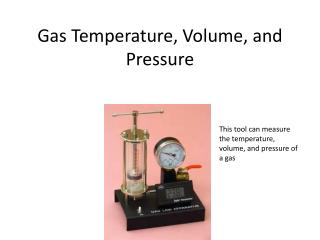DownloadDownload PresentationGas Temperature, Volume, and Pressure

# Gas Temperature, Volume, and Pressure

Télécharger la présentation## Gas Temperature, Volume, and Pressure

- - - - - - - - - - - - - - - - - - - - - - - - - - - E N D - - - - - - - - - - - - - - - - - - - - - - - - - - -
##### Presentation Transcript

1. Gas Temperature, Volume, and Pressure This tool can measure the temperature, volume, and pressure of a gas

2. What is temperature? • All particles in all things are moving at all times, but some faster than others • Temperature measures the average speed of the particles in something Some of the particles move faster or slower than the average, as shown in this diagram

3. Kelvin Temperature Scale • The Kelvin scale of temperature (K) is just like the Celsius scale (⁰C), 0 K is absolute zero. • 0 K=-273 ⁰C • The Kelvin scale, not the Celsius scale, is used when doing math problems This picture shows how the Celsius scale is related to the Kelvin scale

4. Absolute Zero Temperature • Absolute zero (0 K) is the coldest possible temperature. It is the temperature at which all movement stops • Scientists have made temperatures very close to absolute zero, but it is thought that absolute zero can never be reached Absolute Zero is much colder than this

5. Review Boyle’s Law • Boyle’s Law shows how the pressure of a gas changes with volume (or volume with pressure) • P1V1=P2V2

6. Charles’s Law • Charles’s Law shows how the volume of a gas changes with temperature (or temperature with volume) • V1 V2 T1 T2 =

7. Question: The pressure does not change. 2 L 200 K 10 L 1000 K What is the final temperature?

8. The Ideal Gas Law • The Ideal Gas Law combines Boyle’s Law, Charles’s Law, and the number of particles (atoms or molecules) into one equation • (Ideal means “perfect”) • P1V1 P2V2 (n is the number of particles) n1T1 n2T2 =

9. Ideal Gas Law Assumptions • An assumption is something taken as truein a certain situation • The Ideal Gas Law assumes a few things about the particles in a gas • These assumptions are usually very close to being true, but are never completely true • That’s why it’s called the “Ideal” Gas Law instead of the “Real” Gas Law

10. Question: • You are at the top of a mountain where the pressure is 0.5 atm and the temp. is 200 K. You put 2 L of air into a balloon and then take it down the mountain. Along the way, half of the air leaks out of the balloon. At the bottom of the mountain the pressure is 1 atm and the temp. is 300 K. What is the new volume? Answer: 0.75 L